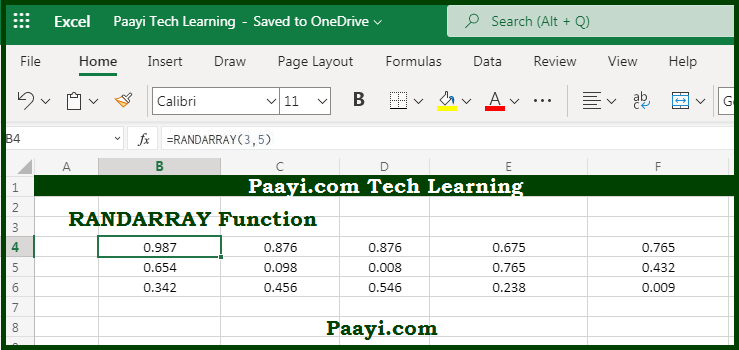# Learn How to Use Microsoft Excel RANDARRAY Function

Written by | 0 Comments | 465 Views

In this article, you will learn how to use the Microsoft Excel RANDARRAY function and its prime function in Microsoft Excel. You will also get to know the Microsoft Excel RANDARRAY function return value and syntax with the help of some examples.

Microsoft Excel RANDARRAY Function

The primary function of the Microsoft Excel RANDARRAY function is to get an array of random numbers. That means with the help of RANDARRAY, you can generate a variety of random numbers in between. The array can be specified by the rows and column arguments, and the values generated can either be decimals or whole numbers.

Return Value of RANDARRAY Function

The return value array of random numbers

Syntax of RANDARRAY Function

=RANDARRAY([rows], [columns], [min], [max], [integer])

Where the arguments:

rows: The number of rows to return, default is 1 (optional).

columns: This is the number of columns to return, default is 1 (optional).

min: This is the minimum value to return, default is 0 (optional).

max: This is the maximum value to return, default is 1, (optional).

integer: This returns the whole numbers, Boolean TRUE or FALSE, default is FALSE (optional).

## How to Use Microsoft Excel RANDARRAY Function?The Microsoft Excel RANDARRAY function is a dynamic array function. The function returns the array of random numbers between 0 and 1. You can determine the size of the array with the help of supplied rows and columns arguments. The value generated by the RANDARRAY function can either be a whole number or decimal value. In case the RANDARRAY returns multiple results, it will spill up in an adjacent cell.